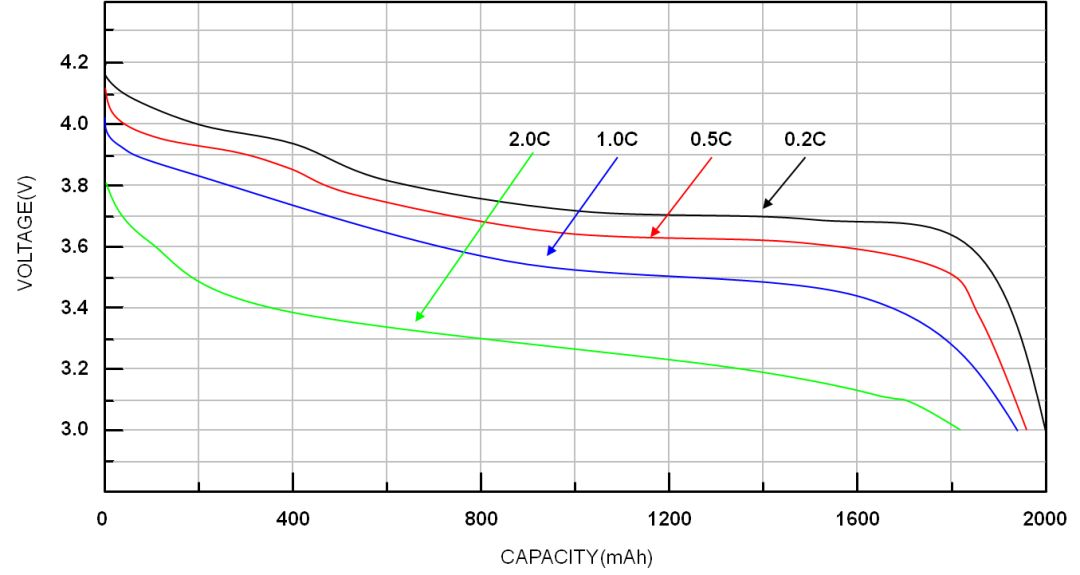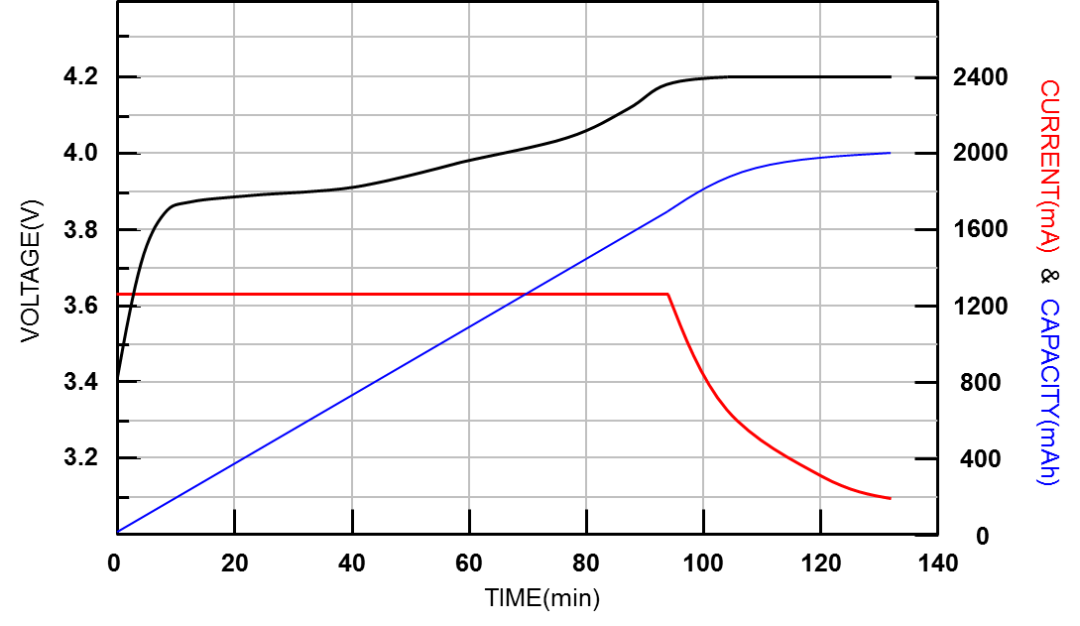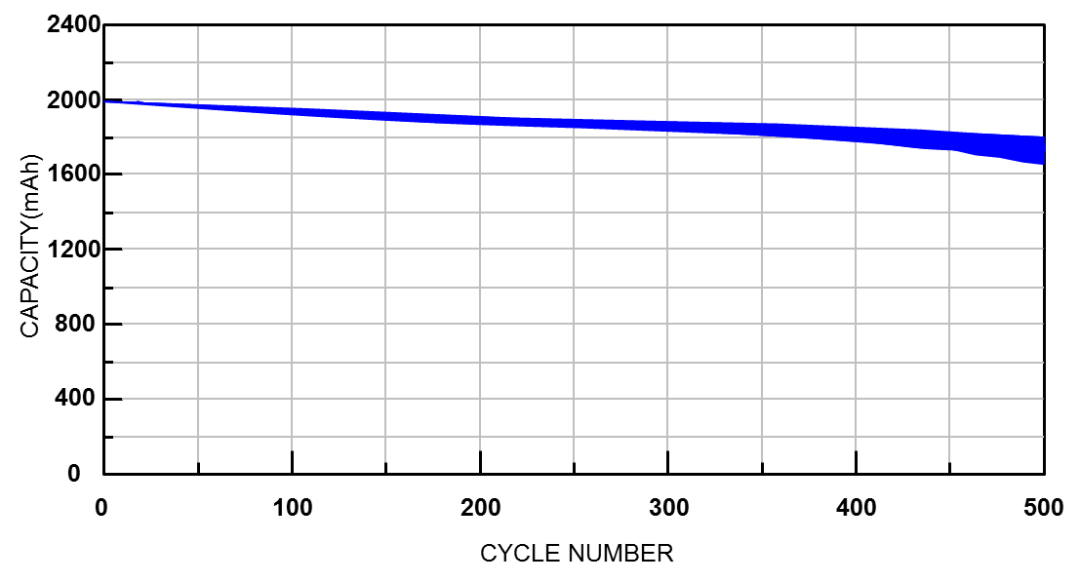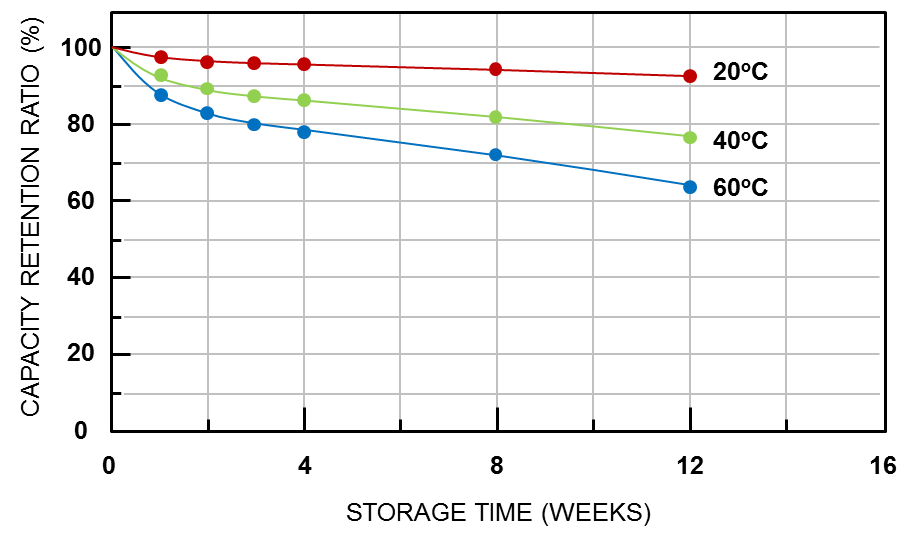# The theory of Lithium charge and discharge & the design of electricity calculation method（1）

1. Introduction to lithium-ion battery

1.1 State of Charge (SOC)

State of charge can be defined as the state of available electric energy in the battery, usually expressed as a percentage. Because the available electric energy varies with the charging and discharging current, temperature and aging phenomenon, the definition of state of charge is also divided into two types: Absolute State-Of-Charge (ASOC) and Relative State-Of-Charge (RSOC).

Generally, the range of relative state of charge is 0% – 100%, while it is 100% when the battery is fully charged and 0% when it is fully discharged. The absolute state of charge is a reference value calculated according to the designed fixed capacity value when the battery is manufactured. The absolute state of charge of a new fully charged battery is 100%; Even if the aging battery is fully charged, it cannot reach 100% under different charging and discharging conditions.

The following figure shows the relationship between voltage and battery capacity at different discharge rates. The higher the discharge rate, the lower the battery capacity. When the temperature is low, the battery capacity will also decrease.Figure 1. Relationship between voltage and capacity under different discharge rates and temperatures

1.2 Max Charging Voltage

The maximum charging voltage is related to the chemical composition and characteristics of the battery. The charging voltage of lithium battery is usually 4.2V and 4.35V, and the voltage values of cathode and anode materials will vary.

1.3 Fully Charged

When the difference between the battery voltage and the maximum charging voltage is less than 100mV and the charging current is reduced to C/10, the battery can be regarded as fully charged. The full charging conditions vary with the characteristics of the battery.

The figure below shows a typical lithium battery charging characteristic curve. When the battery voltage is equal to the maximum charging voltage and the charging current is reduced to C/10, the battery is deemed to be fully chargedFigure 2. Lithium battery charging characteristic curve

1.4 Minimum discharge voltage

The minimum discharge voltage can be defined by the cut-off discharge voltage, which is usually the voltage when the state of charge is 0%. This voltage value is not a fixed value, but changes with load, temperature, aging degree or other factors.

1.5 Full Discharge

When the battery voltage is less than or equal to the minimum discharge voltage, it can be called complete discharge.

1.6 Charge and discharge rate (C-Rate)

The charge-discharge rate is a representation of the charge-discharge current relative to the battery capacity. For example, if you use 1C to discharge for one hour, ideally, the battery will discharge completely. Different charge-discharge rates will result in different usable capacity. Generally, the higher the charge-discharge rate, the smaller the available capacity.

1.7 Cycle life

The number of cycles refers to the number of complete charge and discharge of a battery, which can be estimated by the actual discharge capacity and design capacity. When the accumulated discharge capacity is equal to the design capacity, the number of cycles shall be one. Generally, after 500 charge-discharge cycles, the capacity of the fully charged battery will decrease by 10%~20%.Figure 3. Relationship between cycle times and battery capacity

1.8 Self-discharge

The self-discharge of all batteries will increase with the increase of temperature. Self-discharge is basically not a manufacturing defect, but the characteristics of the battery itself. However, improper treatment in the manufacturing process will also cause the increase of self-discharge. Generally, the self-discharge rate will be doubled when the battery temperature increases by 10 ° C. The self-discharge capacity of lithium-ion batteries is about 1-2% per month, while that of various nickel-based batteries is 10-15% per month.Figure 4. Performance of self-discharge rate of lithium battery at different temperatures

Post time: Feb-07-2023
•#### Phone

•